# Can anyone explain in detail below question

Along a yard 225m long, 26 trees are planted at equal distances , one tree being at each end of the yard. What is the distance between the consecutive trees ? a)8m b)9m c)10m d) 15m

•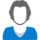• Rohini Shrikant Ohale
• |
• 12 Jul
• |
• 615 Views
• |

text limit upto 4000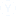• 0

A

• |
• |
• mark
• |
• 12 Jul
• |
• view
• Gaddam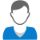• 0

10m

• |
• |
• mark
• |
• 09 Jul
• |
• view
• Dayal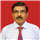• 0

9

• |
• |
• mark
• |
• 08 Jul
• |
• view
• Abhishek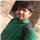• 0

A

• |
• |
• mark
• |
• 08 Jul
• |
• view
• Madhu• 0

8m

• |
• |
• mark
• |
• 08 Jul
• |
• view
• Baseer• 0

9m

• |
• |
• mark
• |
• 08 Jul
• |
• view
• Mohd• 0

Answer : 9The space between 26 consecutive trees would be 25,as it starto from 0.thus 225/25=9

• |
• |
• mark
• |
• 07 Jul
• |
• view
• Sujitha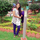• 0

15m

• |
• |
• mark
• |
• 07 Jul
• |
• view
• Pabbati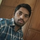• 0

9m

• |
• |
• mark
• |
• 07 Jul
• |
• view
• Vishal• 0

10
because it will start from 0

• |
• |
• mark
• |
• 06 Jul
• |
• view
• Ajay• 0

C)10m

• |
• |
• mark
• |
• 06 Jul
• |
• view
• Venkatraman• 0

since, total trees to be planted=26;
one tree is planted at 0th meter that is at the starting edge of yard
therefore,now 25 trees are to be planted in 225m long yard;
Distance between trees are equal therefore;
Distance=225/25m
Distance=9m

(option) :  b

• |
• |
• mark
• |
• 05 Jul
• |
• view
• Shreyansh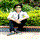• 0

9

• |
• |
• mark
• |
• 05 Jul
• |
• view
• Shipla• 0

• |
• |
• mark
• |
• 05 Jul
• |
• view
• Shaikh• 0

8m

• |
• |
• mark
• |
• 05 Jul
• |
• view
• Gerald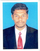• 0

8m

• |
• |
• mark
• |
• 05 Jul
• |
• view
• Ammu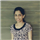• 0

Length of yard is 225 Mtr No of Trees is 26 1 Treee is begining of the Yard (1,2,3,.........225) So, 26-1 = 25 and 25th is the last of the Yard so, 225/25 = 9mtr +------+-------+------+so on 25 Answer is : b) 9 m

• |
• |
• mark
• |
• 05 Jul
• |
• view
• Ajay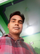• 0

the actual answer should be 8 m.

• |
• |
• mark
• |
• 04 Jul
• |
• view
• Pranjal• 0

Length of yard is 225 Mtr
No of Trees is 26
1 Treee is begining of the Yard (1,2,3,.........225)
So, 26-1 = 25
and 25th is the last of the Yard
so, 225/25 = 9mtr
+------+-------+------+so on 25
Answer is : b) 9 m

• |
• |
• mark
• |
• 04 Jul
• |
• view
• Navin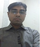• 0

• |
• |
• mark
• |
• 04 Jul
• |
• view
• Sundhar